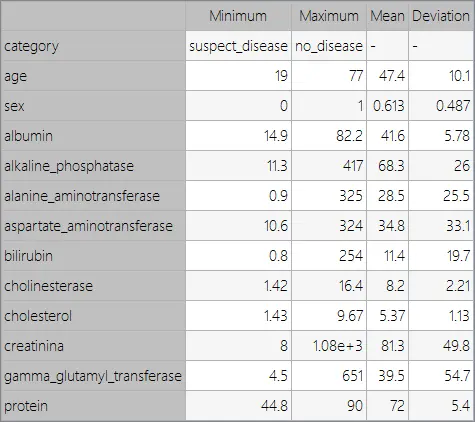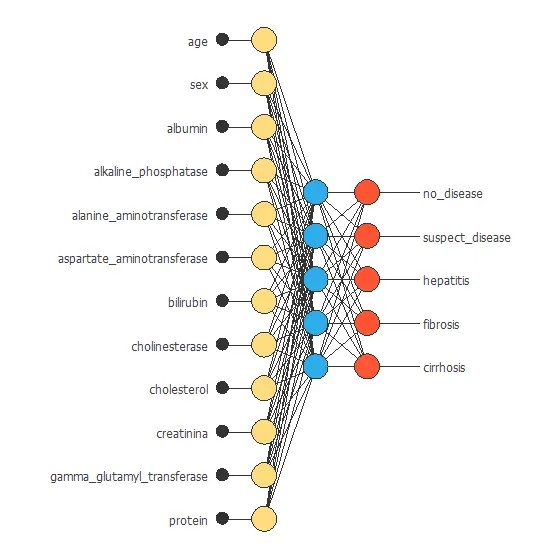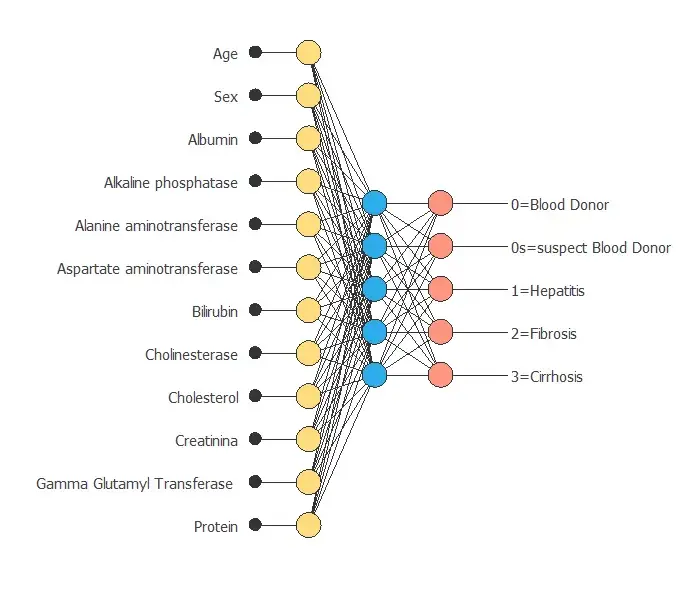This example aims to conclude whether the patient suffers from Hepatitis C, Fibrosis, Cirrhosis, or none of them.

The database used for this study was taken from the Medical University of Hannover, Germany.

### Contents

This example is solved with Neural Designer. To follow it step by step, you can use the free trial.

## 1. Application type

The variable to be predicted is categorical (no disease, suspect disease, hepatitis c, fibrosis, cirrhosis). Therefore, this is a classification project.

The goal here is to model the probability if the patient does not have any disease or suffers from hepatitis, fibrosis, or cirrhosis, conditioned on different tests such as blood analysis or urine tests.

## 2. Data set

The hvcdat.csv file contains the data for this application. In a classification project type, target variables can have five values: no disease, suspect disease, Hepatitis C, Fibrosis, or Cirrhosis. The number of instances (rows) in the data set is 615, and the number of variables (columns) is 13.

The number of input variables, or attributes for each sample, is 12. Nearly all input variables are numeric-valued except one, Sex, which is binary, and most of them represent measurements from blood and urine analysis. The number of target variables is 1 and represents whether the patient suffers from liver disease. The following list summarizes the variables information:

• category(diagnose): No disease, suspect disease, hepatitis c, fibrosis, or cirrhosis.
• age: (0-100). The normal ranges change depending on the age.
• sex: (f or m). The normal ranges change depending on the sex.
• albumin: (normal range 34-54g/L). A blood albumin level below normal may be a sign of kidney disease or liver disease as Hepatitis or Cirrhosis.
• alkaline_phosphatase: (40-129 U/L). It is an alkaline phosphatase test to check for liver disease or liver damage. The main cause or condition that can cause normal levels to rise is liver disease.
• alanine_aminotransferase: (7-55 U/L). Alanine aminotransferase, or ALT is an enzyme found primarily in the liver. An elevated amount indicates liver damage from hepatitis, infection, cirrhosis, liver cancer, or other liver diseases.
• aspartate_aminotransferase: (8-48 U/L). It is an enzyme found primarily in the liver, but also in the muscles. Elevated levels of AST in the blood may indicate hepatitis, cirrhosis, mononucleosis, or other liver diseases.
• bilirubin: (1-12 mg/L). It is a yellowish substance that forms during the normal process of breaking down red blood cells in the body. Lower-than-normal bilirubin levels are generally not a concern. Elevated levels may indicate liver disease or damage.
• cholinesterase: (8-18 U/L). It is a blood test that studies the levels of 2 substances that help the nervous system to function properly. Cholinesterase deficiency causes liver disease, and renal disease…
• cholesterol: (Less than 5,2 mmol/L). Cholesterol is a waxy, fat-like substance found in every cell in your body. A high level could cause carotid artery disease, stroke, and peripheral artery disease…
• creatinina: (61.9-114.9 ??mol/L for m and 53-97.2 ??mol/L for f). It measures the level of creatinine in the blood. High levels of creatinine in the blood and low levels in the urine indicate kidney disease or affecting the function of the kidneys
• gamma_glutamyl_transferase : (from 0 to 30-50 IU/L.). It is an enzyme that indicates cholestasis. The higher the level of GGT, the greater the level of liver damage.
• protein: (Less than 80 mg). It measures the amount of protein in the urine. If protein levels in the urine are elevated, this could indicate kidney damage or another medical problem.

Finally, the use of all instances is set. Note that each instance contains the input and target variables of a different patient.
The data set is divided into training, validation, and testing subsets. 60% of the instances will be assigned for training, 20% for generalization, and 20% for testing. More specifically, 369 samples are used here for training, 123 for selection, and 123 for testing.

Once the data set has been set, we can perform a few related analytics. We check the provided information and make sure that the data is of good quality.

We can calculate the data statistics and draw a table with the minimums, maximums, means, and standard deviations of all variables in the data set. The next table depicts the values.Also, we can calculate the distributions for all variables. The following figure shows a pie chart with the number of instances belonging to each class in the data set.As we can see, no disease people represent 86% of the samples, and the total Hepatitis C, Fibrosis, and Cirrhosis represent approximately 12% of the patients.

We can also calculate the inputs-targets correlations to see which signals better define each factor. The following chart illustrates the dependency of the target category with the input variables of the data set.Here, the most correlated variables with Hepatitis C are aspartate aminotransferase, gamma glutamyl transferase, and alanine aminotransferase.

## 3. Neural network

The second step is to set a neural network representing the classification function. For this class of applications, the neural network is composed of:

• Scaling layer.
• Perceptron layers.
• Probabilistic layer.

The scaling layer contains the statistics on the inputs calculated from the data file and the method for scaling the input variables. Here the minimum-maximum method has been set. Nevertheless, the mean-standard deviation method would produce very similar results.

The number of perceptron layers is 1. This perceptron layer has 12 inputs and 5 neurons.

The probabilistic layer uses the softmax probabilistic method.

The following figure is a graphical representation of this neural network for liver disease diagnosis.## 4. Training strategy

The procedure used to carry out the learning process is called a training strategy. The training strategy is applied to the neural network to obtain the best possible performance. The type of training is determined by how the adjustment of the parameters in the neural network takes place. The fourth step is composed of two terms:

• A loss index.
• An optimization algorithm.

The loss index is the cross entropy error with L1 regularization.

The learning problem can be stated as finding a neural network that minimizes the loss index. That is a neural network that fits the data set (error term) and does not oscillate (regularization term).

The optimization algorithm that we use is the quasi-Newton method. This is also the standard optimization algorithm for this type of problem.The following chart shows how the error decreases with the iterations during the training process. This is a sign of convergence.
The final training and selection errors are training error = 0.0553 WSE and selection error = 0.0557 WSE, respectively.

## 5. Model selection

The objective of model selection is to improve the generalization capabilities of the neural network or, in other words, to reduce the selection error.

Since the selection error that we have achieved so far is very small (0.0557 NSE), we don’t need to apply order selection or input selection here.

## 6. Testing analysis

Once the model is trained, we perform a testing analysis to validate its prediction capacity. We use a subset of data that has not been used before, the testing instances.

The next table shows the confusion matrix for our problem. The confusion matrix represents the real classes and the predicted classes’ columns for the testing data.

Predicted no_disease Predicted suspect_disease Predicted hepatitis_c Predicted fibrosis Predicted cirrhosis
Real no_disease 107 (87%) 1(0.813%) 0 1(0.813%) 0
Real suspect_disease 0 0 0 0 0
Real hepatitis_c 5(4.07%) 0 1(0.813%) 1(0.813%) 1(0.813%)
Real fibrosis 0 0 1(0.813%) 2(1.63%) 0
Real cirrhosis 1(0.813%) 0 0 0 2(1.63%)

As we can see, the number of instances that the model can correctly predict is 123 (92%), while it approximately misclassifies only 11 (8%). This shows that our predictive model has excellent classification accuracy, and the biggest confusion is predicting no disease when the patient suffers from hepatitis c.

## 7. Model deployment

Once the neural network’s generalization performance has been tested, the neural network can be saved for future use in the so-called model deployment mode.

We can diagnose new patients by calculating the neural network outputs. For that, we need to know the input variables for them. An example is the following:

We can export the mathematical expression of the neural network to the hospital’s software to facilitate the work of the doctor. This expression is listed below.

scaled_age = age*(1+1)/(77-(19))-19*(1+1)/(77-19)-1;
scaled_sex = (sex-(0.6130080223))/0.4874579906;
scaled_albumin = albumin*(1+1)/(82.19999695-(14.89999962))-14.89999962*(1+1)/(82.19999695-14.89999962)-1;
scaled_alkaline_phosphatase = alkaline_phosphatase*(1+1)/(416.6000061-(11.30000019))-11.30000019*(1+1)/(416.6000061-11.30000019)-1;
scaled_alanine_aminotransferase = alanine_aminotransferase*(1+1)/(325.2999878-(0.8999999762))-0.8999999762*(1+1)/(325.2999878-0.8999999762)-1;
scaled_aspartate_aminotransferase = aspartate_aminotransferase*(1+1)/(324-(10.60000038))-10.60000038*(1+1)/(324-10.60000038)-1;
scaled_bilirubin = bilirubin*(1+1)/(254-(0.8000000119))-0.8000000119*(1+1)/(254-0.8000000119)-1;
scaled_cholinesterase = cholinesterase*(1+1)/(16.40999985-(1.419999957))-1.419999957*(1+1)/(16.40999985-1.419999957)-1;
scaled_cholesterol = cholesterol*(1+1)/(9.670000076-(1.429999948))-1.429999948*(1+1)/(9.670000076-1.429999948)-1;
scaled_creatinina = creatinina*(1+1)/(1079.099976-(8))-8*(1+1)/(1079.099976-8)-1;
scaled_gamma_glutamyl_transferase = gamma_glutamyl_transferase*(1+1)/(650.9000244-(4.5))-4.5*(1+1)/(650.9000244-4.5)-1;
scaled_protein = protein*(1+1)/(90-(44.79999924))-44.79999924*(1+1)/(90-44.79999924)-1;

perceptron_layer_0_output_0 = sigma[ 0.000687571 + (scaled_age*-0.000107975)+ (scaled_sex*-0.000354215)+ (scaled_albumin*0.000200821)+ (scaled_alkaline_phosphatase*5.53142e-06)+ (scaled_alanine_aminotransferase*-0.000204123)+ (scaled_aspartate_aminotransferase*-0.000175011)+ (scaled_bilirubin*0.000402467)+ (scaled_cholinesterase*-6.37448e-05)+ (scaled_cholesterol*-0.000240513)+ (scaled_creatinina*0.000516883)+ (scaled_gamma_glutamyl_transferase*-5.56635e-05)+ (scaled_protein*0.00018629) ];
perceptron_layer_0_output_1 = sigma[ -0.00100031 + (scaled_age*-0.000225761)+ (scaled_sex*0.000388395)+ (scaled_albumin*0.00128129)+ (scaled_alkaline_phosphatase*9.56258e-05)+ (scaled_alanine_aminotransferase*0.000165274)+ (scaled_aspartate_aminotransferase*0.000264668)+ (scaled_bilirubin*0.000126936)+ (scaled_cholinesterase*0.000348544)+ (scaled_cholesterol*8.74069e-05)+ (scaled_creatinina*0.000187206)+ (scaled_gamma_glutamyl_transferase*4.68866e-05)+ (scaled_protein*0.000123546) ];
perceptron_layer_0_output_2 = sigma[ -0.000135561 + (scaled_age*0.00104903)+ (scaled_sex*-0.000125211)+ (scaled_albumin*0.000365379)+ (scaled_alkaline_phosphatase*0.000471019)+ (scaled_alanine_aminotransferase*0.000246482)+ (scaled_aspartate_aminotransferase*-0.000117056)+ (scaled_bilirubin*0.000598315)+ (scaled_cholinesterase*0.000453945)+ (scaled_cholesterol*-0.000645688)+ (scaled_creatinina*-0.000144883)+ (scaled_gamma_glutamyl_transferase*1.79297e-05)+ (scaled_protein*0.000202182) ];
perceptron_layer_0_output_3 = sigma[ -3.94009e-05 + (scaled_age*0.000279269)+ (scaled_sex*-0.00126496)+ (scaled_albumin*-0.00015788)+ (scaled_alkaline_phosphatase*0.000714173)+ (scaled_alanine_aminotransferase*0.000159489)+ (scaled_aspartate_aminotransferase*-0.000126977)+ (scaled_bilirubin*-0.000248145)+ (scaled_cholinesterase*0.000231954)+ (scaled_cholesterol*-0.000381098)+ (scaled_creatinina*0.000388452)+ (scaled_gamma_glutamyl_transferase*-2.25984e-05)+ (scaled_protein*-2.42171e-05) ];
perceptron_layer_0_output_4 = sigma[ 0.531863 + (scaled_age*0.000279123)+ (scaled_sex*0.000294663)+ (scaled_albumin*0.000832503)+ (scaled_alkaline_phosphatase*-0.0035456)+ (scaled_alanine_aminotransferase*-0.000726132)+ (scaled_aspartate_aminotransferase*-0.143645)+ (scaled_bilirubin*-0.0693214)+ (scaled_cholinesterase*-0.000138161)+ (scaled_cholesterol*0.000344551)+ (scaled_creatinina*-0.210249)+ (scaled_gamma_glutamyl_transferase*-0.0841537)+ (scaled_protein*-0.00010063) ];

probabilistic_layer_combinations_0 = 1.99571 +0.00017129*perceptron_layer_0_output_0 +0.000294192*perceptron_layer_0_output_1 +0.000545024*perceptron_layer_0_output_2 +1.71061e-05*perceptron_layer_0_output_3 +1.23961*perceptron_layer_0_output_4
probabilistic_layer_combinations_1 = 2.43231 +8.26751e-06*perceptron_layer_0_output_0 -0.000394384*perceptron_layer_0_output_1 +0.000839893*perceptron_layer_0_output_2 -0.000321675*perceptron_layer_0_output_3 +1.06731*perceptron_layer_0_output_4
probabilistic_layer_combinations_2 = 2.85722 +0.000411359*perceptron_layer_0_output_0 +0.000485672*perceptron_layer_0_output_1 +9.30878e-05*perceptron_layer_0_output_2 -7.38007e-05*perceptron_layer_0_output_3 +0.789123*perceptron_layer_0_output_4
probabilistic_layer_combinations_3 = 1.99249 +0.000452945*perceptron_layer_0_output_0 +0.00107266*perceptron_layer_0_output_1 +0.000121336*perceptron_layer_0_output_2 -0.000247549*perceptron_layer_0_output_3 +1.1734*perceptron_layer_0_output_4
probabilistic_layer_combinations_4 = 2.01512 +0.000355189*perceptron_layer_0_output_0 +0.000699017*perceptron_layer_0_output_1 -0.000694358*perceptron_layer_0_output_2 +0.000623235*perceptron_layer_0_output_3 +0.226457*perceptron_layer_0_output_4

no_disease = 1.0/(1.0 + exp(-probabilistic_layer_combinations_0);
suspect_disease = 1.0/(1.0 + exp(-probabilistic_layer_combinations_1);
hepatitis = 1.0/(1.0 + exp(-probabilistic_layer_combinations_2);
fibrosis = 1.0/(1.0 + exp(-probabilistic_layer_combinations_3);
cirrhosis = 1.0/(1.0 + exp(-probabilistic_layer_combinations_4);


## References

• The data for this problem has been taken from the UCI Machine Learning Repository.
• Lichtinghagen R et al. J Hepatol 2013; 59: 236-42.
• Hoffmann G et al. Using machine learning techniques to generate laboratory diagnostic pathways a case study. J Lab Precis Med 2018; 3: 58-67.